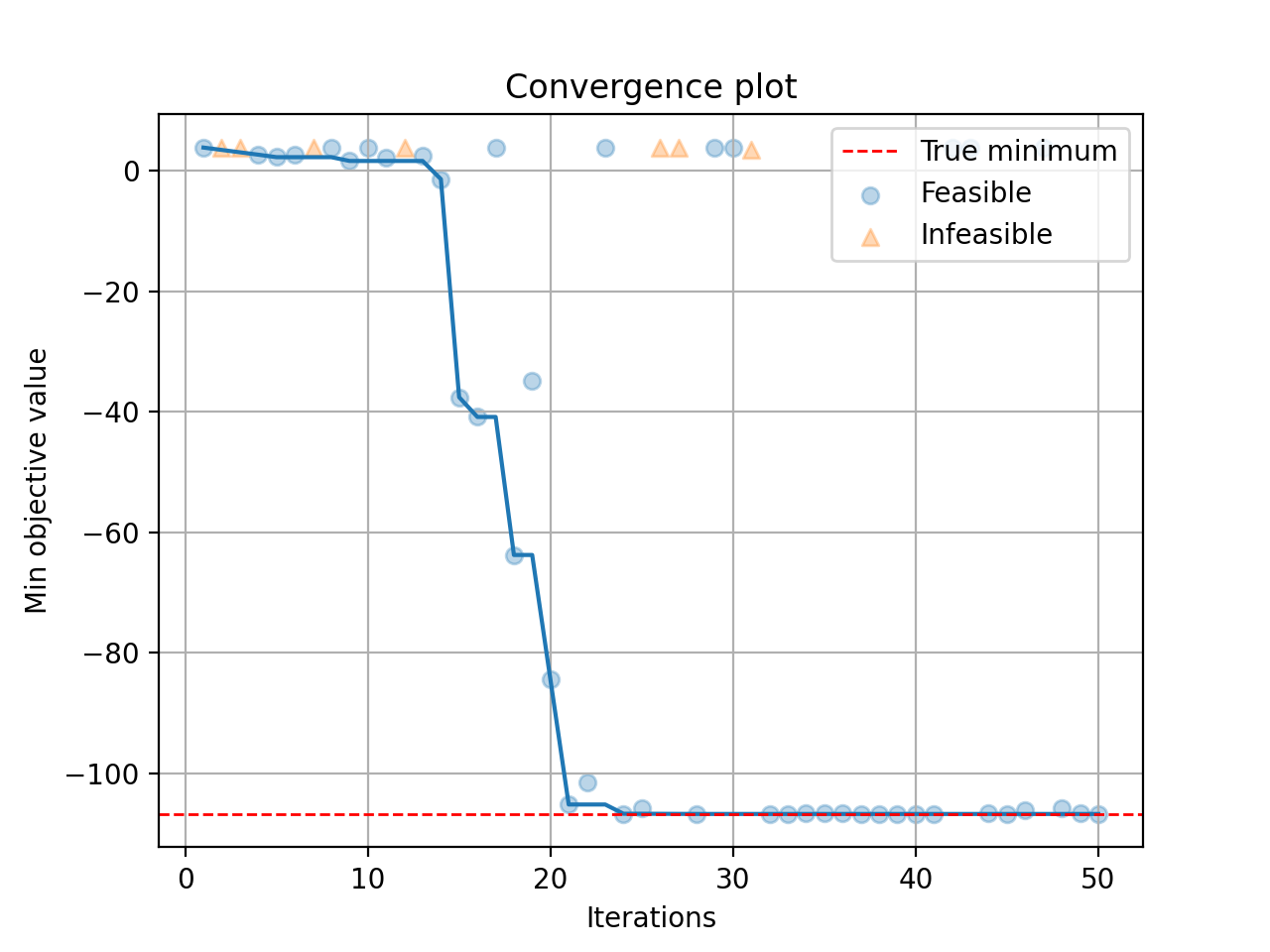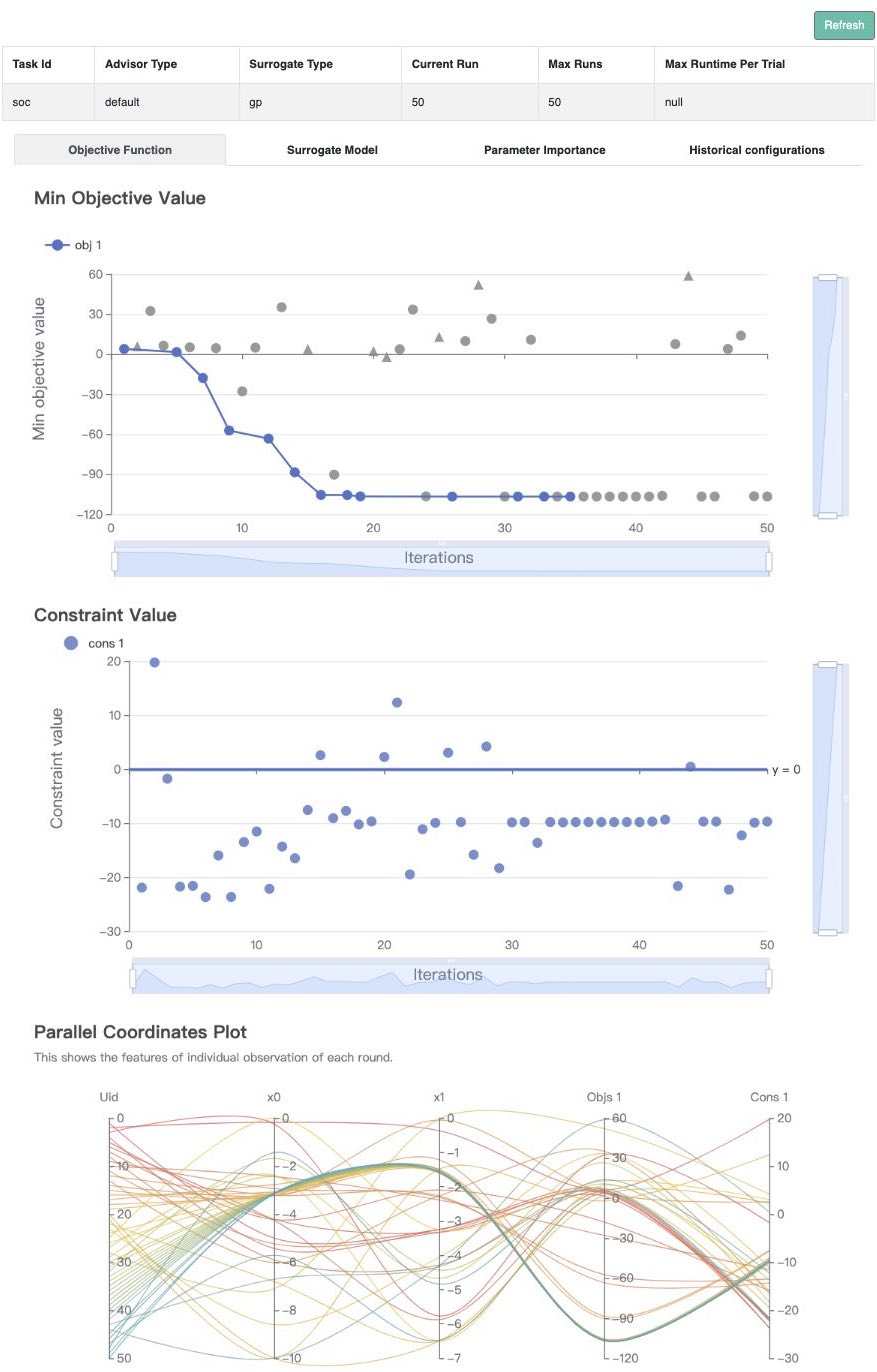# Single-Objective with Constraints#

In this tutorial, we will introduce how to optimize a constrained problem with OpenBox.

## Problem Setup#

First, define search space and define objective function to minimize. Here we use the constrained Mishra function.

import numpy as np
from openbox import space as sp

def mishra(config: sp.Configuration):
X = np.array([config['x%d' % i] for i in range(2)])
x, y = X, X
t1 = np.sin(y) * np.exp((1 - np.cos(x))**2)
t2 = np.cos(x) * np.exp((1 - np.sin(y))**2)
t3 = (x - y)**2

result = dict()
result['objectives'] = [t1 + t2 + t3, ]
result['constraints'] = [np.sum((X + 5)**2) - 25, ]
return result

params = {
'float': {
'x0': (-10, 0, -5),
'x1': (-6.5, 0, -3.25)
}
}
space = sp.Space()
sp.Real(name, *para) for name, para in params['float'].items()
])


After evaluation, the objective function returns a dict (Recommended). The result dictionary should contain:

• 'objectives': A list/tuple of objective values (to be minimized). In this example, we have only one objective so the tuple contains a single value.

• 'constraints': A list/tuple of constraint values. Non-positive constraint values (“<=0”) imply feasibility.

## Optimization#

After defining the search space and the objective function, we can run the optimization process as follows:

from openbox import Optimizer

opt = Optimizer(
mishra,
space,
num_constraints=1,
num_objectives=1,
surrogate_type='gp',
acq_optimizer_type='random_scipy',
max_runs=50,
# Have a try on the new HTML visualization feature!
# visualization='advanced',   # or 'basic'. For 'advanced', run 'pip install "openbox[extra]"' first
# auto_open_html=True,        # open the visualization page in your browser automatically
)
history = opt.run()


Here we create a Optimizer instance, and pass the objective function and the search space to it. The other parameters are:

• num_objectives=1 and num_constraints=1 indicate that our function returns a single value with one constraint.

• max_runs=50 means the optimization will take 50 rounds (optimizing the objective function 50 times).

• task_id is set to identify the optimization process.

• visualization: 'none', 'basic' or 'advanced'. See HTML Visualization.

• auto_open_html: whether to open the visualization page in your browser automatically. See HTML Visualization.

Then, opt.run() is called to start the optimization process.

## Visualization#

After the optimization, opt.run() returns the optimization history. Or you can call opt.get_history() to get the history. Then, call print(history) to see the result:

history = opt.get_history()
print(history)

+-------------------------+---------------------+
| Parameters              | Optimal Value       |
+-------------------------+---------------------+
| x0                      | -3.172421           |
| x1                      | -1.506397           |
+-------------------------+---------------------+
| Optimal Objective Value | -105.72769850551406 |
+-------------------------+---------------------+
| Num Configs             | 50                  |
+-------------------------+---------------------+


Call history.plot_convergence() to visualize the optimization process:

import matplotlib.pyplot as plt
history.plot_convergence(true_minimum=-106.7645367)
plt.show()(New Feature!) Call history.visualize_html() to visualize the optimization process in an HTML page. For show_importance and verify_surrogate, run pip install "openbox[extra]" first. See HTML Visualization for more details.

history.visualize_html(open_html=True, show_importance=True,
verify_surrogate=True, optimizer=opt)